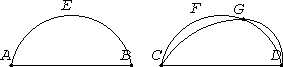# Proposition 24

Similar segments of circles on equal straight lines equal one another.

Let AEB and CFD be similar segments of circles on equal straight lines AB and CD.

I say that the segment AEB equals the segment CFD.For, if the segment AEB is superposed on CFD, and if the point A is placed on C and the straight line AB on CD, then the point B coincides with the point D, because AB equals CD, and, AB coinciding with CD, the segment AEB also coincides with CFD.

For, if the straight line AB coincides with CD but the segment AEB does not coincide with CFD, then it either falls within it, or outside it, or it cuts across it, as CGD, and a circle cuts a circle at more points than two, which is impossible.

C.N.4

Therefore, if the straight line AB is superposed on CD, then the segment AEB does not fail to coincide with CFD also, therefore it coincides with it and equals it.

Therefore similar segments of circles on equal straight lines equal one another.

Q.E.D.

## Guide

The proof here uses the method of superposition which was also used for I.4 and I.8.

This proposition is used in III.26.